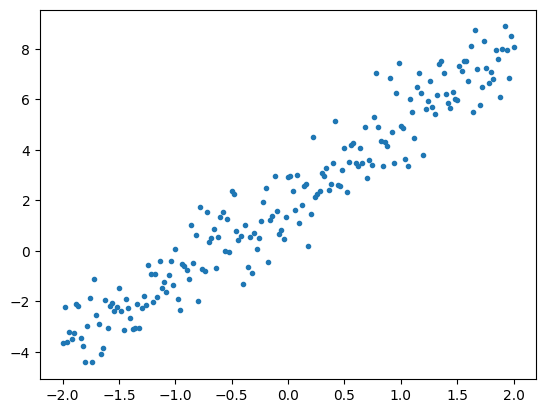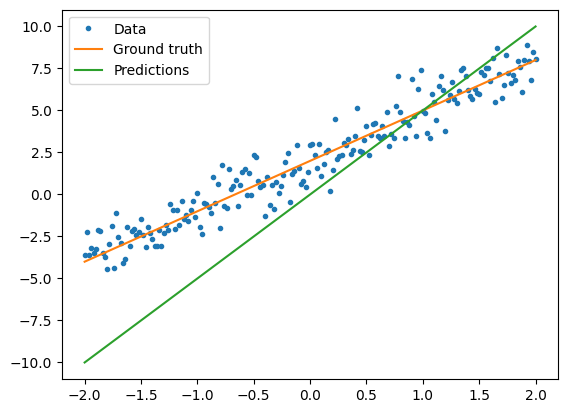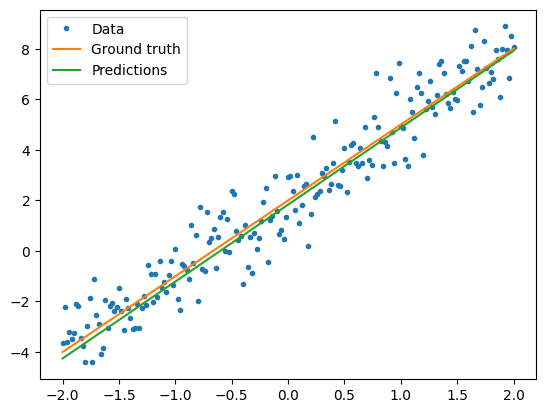基本训练循环

TensorFlow 同样具有更高层次的神经网络应用程序编程接口 tf.Keras。它提供有效的抽象来减少代码量。然而，这篇教程中，您将使用基础类。

创建

import tensorflow as tf

解决机器学习问题

• 获得训练数据。
• 定义模型。
• 定义损失函数。
• 遍历训练数据，从目标值计算损失。
• 计算该损失的梯度，并使用optimizer调整变量以适合数据。
• 计算结果。

数据

TensorFlow中几乎每个输入数据都是由张量表示，并且通常是向量。监督学习中，输出(即想到预测值)同样是个张量。

# 实际的线
TRUE_W = 3.0
TRUE_B = 2.0

NUM_EXAMPLES = 1000

# 随机向量x
x = tf.random.normal(shape=[NUM_EXAMPLES])

# 生成噪声
noise = tf.random.normal(shape=[NUM_EXAMPLES])

# 计算y
y = x * TRUE_W + TRUE_B + noise
# 绘制所有的数据
import matplotlib.pyplot as plt

plt.scatter(x, y, c="b")
plt.show()定义模型

class MyModel(tf.Module):
def __init__(self, **kwargs):
super().__init__(**kwargs)
# 初始化权重值为5.0，偏差值为0.0
# 实际项目中，应该随机初始化
self.w = tf.Variable(5.0)
self.b = tf.Variable(0.0)

def __call__(self, x):
return self.w * x + self.b

model = MyModel()

# 列出变量tf.modules的内置变量聚合
print("Variables:", model.variables)

# 验证模型是否有效
assert model(3.0).numpy() == 15.0
Variables: (<tf.Variable 'Variable:0' shape=() dtype=float32, numpy=0.0>, <tf.Variable 'Variable:0' shape=() dtype=float32, numpy=5.0>)

定义损失函数

# 计算整个批次的单个损失值
def loss(target_y, predicted_y):
return tf.reduce_mean(tf.square(target_y - predicted_y))

plt.scatter(x, y, c="b")
plt.scatter(x, model(x), c="r")
plt.show()

print("Current loss: %1.6f" % loss(model(x), y).numpy())Current loss: 9.350621

定义训练循环

• 发送一批输入值，通过模型生成输出值
• 通过比较输出值与输出（标签），来计算损失值
• 使用这些梯度优化变量

# 给定一个可调用的模型，输入，输出和学习率...
def train(model, x, y, learning_rate):

current_loss = loss(y, model(x))

dw, db = t.gradient(current_loss, [model.w, model.b])

# 减去由学习率缩放的梯度
model.w.assign_sub(learning_rate * dw)
model.b.assign_sub(learning_rate * db)

model = MyModel()

# 收集W值和b值的历史记录以供以后绘制
Ws, bs = [], []
epochs = range(10)

# 定义用于训练的循环
def training_loop(model, x, y):

for epoch in epochs:
# 用单个大批次处理更新模型
train(model, x, y, learning_rate=0.1)

# 在更新之前进行跟踪
Ws.append(model.w.numpy())
bs.append(model.b.numpy())
current_loss = loss(y, model(x))

print("Epoch %2d: W=%1.2f b=%1.2f, loss=%2.5f" %
(epoch, Ws[-1], bs[-1], current_loss))
print("Starting: W=%1.2f b=%1.2f, loss=%2.5f" %
(model.w, model.b, loss(y, model(x))))

# 开始训练
training_loop(model, x, y)

# 绘制
plt.plot(epochs, Ws, "r",
epochs, bs, "b")

plt.plot([TRUE_W] * len(epochs), "r--",
[TRUE_B] * len(epochs), "b--")

plt.legend(["W", "b", "True W", "True b"])
plt.show()
Starting: W=5.00 b=0.00, loss=9.35062
Epoch  0: W=4.58 b=0.41, loss=6.25026
Epoch  1: W=4.25 b=0.74, loss=4.30416
Epoch  2: W=3.98 b=0.99, loss=3.08259
Epoch  3: W=3.77 b=1.20, loss=2.31581
Epoch  4: W=3.61 b=1.36, loss=1.83451
Epoch  5: W=3.48 b=1.49, loss=1.53239
Epoch  6: W=3.37 b=1.59, loss=1.34275
Epoch  7: W=3.29 b=1.67, loss=1.22371
Epoch  8: W=3.22 b=1.73, loss=1.14900
Epoch  9: W=3.17 b=1.79, loss=1.10209# 可视化训练后的模型如何执行
plt.scatter(x, y, c="b")
plt.scatter(x, model(x), c="r")
plt.show()

print("Current loss: %1.6f" % loss(model(x), y).numpy())Current loss: 1.102094

使用Keras完成相同的解决方案

class MyModelKeras(tf.keras.Model):
def __init__(self, **kwargs):
super().__init__(**kwargs)
# 初始化权重为5.0，偏差为0.0
# 实际中应该随机初始化该值
self.w = tf.Variable(5.0)
self.b = tf.Variable(0.0)

def __call__(self, x, **kwargs):
return self.w * x + self.b

keras_model = MyModelKeras()

# 使用Keras模型重新进行循环训练
training_loop(keras_model, x, y)

# 您同样可以使用Keras内置的功能保存检查点（checkpoint）
keras_model.save_weights("my_checkpoint")
Epoch  0: W=4.58 b=0.41, loss=6.25026
Epoch  1: W=4.25 b=0.74, loss=4.30416
Epoch  2: W=3.98 b=0.99, loss=3.08259
Epoch  3: W=3.77 b=1.20, loss=2.31581
Epoch  4: W=3.61 b=1.36, loss=1.83451
Epoch  5: W=3.48 b=1.49, loss=1.53239
Epoch  6: W=3.37 b=1.59, loss=1.34275
Epoch  7: W=3.29 b=1.67, loss=1.22371
Epoch  8: W=3.22 b=1.73, loss=1.14900
Epoch  9: W=3.17 b=1.79, loss=1.10209

keras_model = MyModelKeras()

# 编译设置培训参数
keras_model.compile(
# 默认情况下，fit()调用tf.function()。
# Debug时你可以关闭这一功能，但是现在是打开的。
run_eagerly=False,

# 使用内置的优化器，配置为对象
optimizer=tf.keras.optimizers.SGD(learning_rate=0.1),

# Keras内置MSE
# 您也可以使用损失函数像上面一样进行定义
loss=tf.keras.losses.mean_squared_error,
)

Kerasfit期望批处理数据或完整的数据集作为NumPy数组。 NumPy数组分为多个批次，默认批次大小为32。

print(x.shape)
keras_model.fit(x, y, epochs=10, batch_size=1000)
1000
Epoch 1/10
1/1 [==============================] - 0s 183ms/step - loss: 9.3506
Epoch 2/10
1/1 [==============================] - 0s 2ms/step - loss: 6.2503
Epoch 3/10
1/1 [==============================] - 0s 1ms/step - loss: 4.3042
Epoch 4/10
1/1 [==============================] - 0s 2ms/step - loss: 3.0826
Epoch 5/10
1/1 [==============================] - 0s 1ms/step - loss: 2.3158
Epoch 6/10
1/1 [==============================] - 0s 2ms/step - loss: 1.8345
Epoch 7/10
1/1 [==============================] - 0s 2ms/step - loss: 1.5324
Epoch 8/10
1/1 [==============================] - 0s 2ms/step - loss: 1.3428
Epoch 9/10
1/1 [==============================] - 0s 2ms/step - loss: 1.2237
Epoch 10/10
1/1 [==============================] - 0s 2ms/step - loss: 1.1490
<tensorflow.python.keras.callbacks.History at 0x7f9aa9de9828>

[]
[]